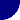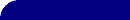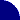# Explaining a Constant Phase Element (CPE)RESOURCES > EIS > CPE > EXPLANATIONS

## What Causes a CPE?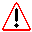In his textbook, Macdonald ( Ref 1 ) points out that even though a particular theory may not give exactly CPE behavior, very often CPE behavior will fit experimental data so well that the deviations are totally masked by experimental noise and uncertainties. This is increasingly true as the complexity of a circuit model grows. In short, a CPE can provide a useful modeling element, even if the true nature of the system is unknown.

###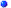Surface Roughness

One physical explanation put forth for CPE behavior is electrode roughness. For a rough, fractal, surface, the fractal dimension (D) of the surface is between 2 and 3: Translated, this means that the surface fills between 2 dimensions (i.e., it's absolutely flat) and 3 dimensions (i.e., the surface fills three dimensions, branching every-which-way through space, and resembling a porous cube.) It has been shown ( Ref 2 ) that for these electrodes, the interfacial impedance (electron transfer or double layer capacitance) is modified by an exponent, n = 1/(D-1). For a smooth surface the fractal dimension (D) is 2.0 and n=1: The impedance is unchanged. For a highly contorted surface (D=3), and n=0.5.

For a great many real metal or solid electrodes, the measured impedance in the double-layer region (no faradaic current) follows a power law, such as that for the CPE, with a value of n between 0.9 and 1.0. The phase angle of this "capacitance" is not 90° , but is given by (n*90°). The observed angle is something between 80° (n=0.89) and 90° (n=1.0). When this "capacitance" is in parallel with a charge-transfer resistance (R), the Nyquist plot is the arc of a circle, but with the center of the circle blow the x-axis: It is the "depressed semi-circle" shown in the figure on the previous page. This behavior is not seen on a mercury electrode because, as a liquid, it is atomically smooth!

###A Distribution of Reaction Rates

Another explanation is inhomogeneous reaction rates on a surface. This might be seen at polycrystalline metal surfaces or carbon electrodes with a distribution of active sites (with varying activation energies) on the surface. A recent article ( Ref 3 ) shows that for a glassy carbon electrode, the CPE exponent correlates with the fraction of exposed edge plane orientation, not with the fractal dimension of the surface. Mercury, which does not show CPE behavior, is also atomically homogeneous, unlike polycrystalline metals.

This explanation is explored further in the discussion of the ZARC circuit element

###Varying Thickness or Composition

A third possible explanation may be varying thickness or composition of a coating. For example, if the bulk conductivity of a coating changes with distance through the coating (see Ref 4), then the resultant impedance spectrum can closely approximate that of a CPE.

###Non-uniform Current Distribution

At the 2004 EIS Symposium, Tribollet ( Ref 5 ) presented a paper in which he looked at the EIS of a Mg alloy in dilute Na2SO4. The impedance of the whole electrode was measured in the traditional way and the spectrum above 1 Hz was fit to a Randles Cell containing a CPE with an n-value of 0.91.

The local impedance was also measured by placing a sub-mm current probe a short distance over the electrode.  The local impedance was measured as a function of the distance along the radius of the electrode.  Near the center, the n-value for the CPE was 1.0, indicating a true capacitance.  However, at the edge of the electrode, the n-value for the CPE was 0.83!  We would expect the current density to be fairly homogeneous near the center of the electrode, and normal to the surface.  Near the edge, the current density will certainly be perturbed by "edge effects."  Current flow will also not be perfectly normal to the surface. Both effects are likely to change the n-value for the CPE.

Another publication "The RC time 'constant' at a disk electrode" ( Ref 6 ) discusses the same phenomenon, but from a slightly different perspective. Oldham showed that the local RC time constant (uncompensated- or solution-resistance times double layer capacitance) varies from 0 to 2RC over the disk.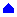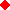Did you find this information helpful? We'd like to know !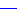REFERENCES(1) "Impedance Spectroscopy", JR Macdonald, ed., John Wiley, 1987.  Sect. 2.2.3.4.(2) "Tafel current at fractal electrodes. Connection with admittance spectra," WH Mulder, JH Sluyters, T Pajkossy, I Nyikos, J. Electroanal. Chem.285 (1990) 103.(3) "An investigation of the capacitance dispersion on the fractal carbon electrode with edge and basal orientations,"  C-H Kim, S-I Pyun, J-H Kim, Electrochimica Acta, 48 (2003) 3455.(4) "The evaluation of experimental dielectric data of barrier coatings by means of different models," CA Schiller, W Strunz, Electrochimica Acta, 46 (2001) 3619.(5) "CPE Analysis by Local Impedance Analysis", J-B Jorcin, ME Orazem, N Pebere, B Tribollet,   Electrochimica Acta, 51 (2006) 1473-1479. Paper presented at EIS Symposium, Coco Beach, FL, June 2004.(6) "The RC time 'constant' at a disk electrode", KB Oldham, Electrochem. Communications, 6 (2004) 210-214.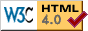© Copyright 2003-2009 Research Solutions & Resources LLC